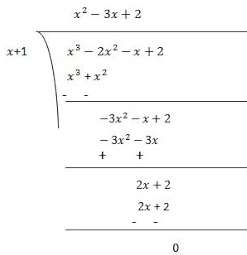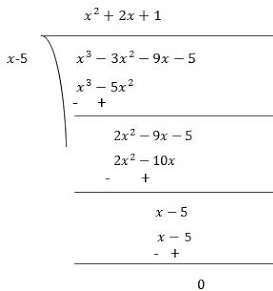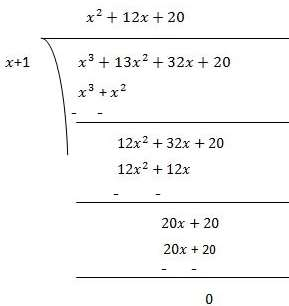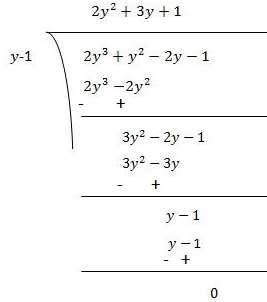Courses

# NCERT Solutions Chapter 2 - Polynomials (II), Class 9, Maths Class 9 Notes | EduRev

Created by: Indu Gupta

## Class 9 : NCERT Solutions Chapter 2 - Polynomials (II), Class 9, Maths Class 9 Notes | EduRev

The document NCERT Solutions Chapter 2 - Polynomials (II), Class 9, Maths Class 9 Notes | EduRev is a part of Class 9 category.
All you need of Class 9 at this link: Class 9

Exercise 2.4

1. Determine which of the following polynomials has (x + 1) a factor:
(i) x3x2x + 1

(ii) x4x3x2x + 1
(iii) x4 + 3x3 + 3x2x + 1

(iv) x3x2 - (2 + √2)x + √2

(i) If (x + 1) is a factor of p(x) = x3x2x + 1, p(-1) must be zero.
Here, p(x) = x3x2x + 1
p(-1) = (-1)3 + (-1)2 + (-1) + 1
= -1 + 1 - 1 + 1 = 0
Therefore, x + 1 is a factor of this polynomial

(ii) If (x + 1) is a factor of p(x) = x4x3x2x + 1, p(-1) must be zero.
Here, p(x) = x4x3x2x + 1
p(-1) = (-1)4 + (-1)3 + (-1)2 + (-1) + 1
= 1 - 1 + 1 - 1 + 1 = 1

As, p(-1) ≠ 0
Therefore, x + 1 is not a factor of this polynomial

(iii)If (x + 1) is a factor of polynomial p(x) = x4 + 3x3 + 3x2x + 1, p(- 1) must be 0.
p(-1) = (-1)4 + 3(-1)3 + 3(-1)2 + (-1) + 1
= 1 - 3 + 3 - 1 + 1 = 1
As, p(-1) ≠ 0
Therefore, x + 1 is not a factor of this polynomial.

(iv) If (x + 1) is a factor of polynomial

p(x) = x3x2 - (2 + √2)x + √2, p(- 1) must be 0.

p(-1) =  (-1)3 -  (-1)2 -  (2 + √2) (-1) + √2
= -1 - 1 + 2 + √2 + √2
=2√2
As, p(-1) ≠ 0
Therefore,, x + 1 is not a factor of this polynomial.

2. Use the Factor Theorem to determine whether g(x) is a factor of p(x) in each of the following cases:
(i) p(x) = 2x3x2 - 2x - 1, g(x) = x + 1
(ii) p(x) = x3 + 3x2 + 3x + 1, g(x) = x + 2
(iii) p(x) = x3 - 4 x2x + 6, g(x) = x - 3

(i) If g(x) = x + 1 is a factor of given polynomial p(x), p(- 1) must be zero.
p(x) = 2x3x2 - 2x - 1
p(- 1) = 2(-1)3 + (-1)2 - 2(-1) - 1
= 2(- 1) + 1 + 2 - 1 = 0
Hence, g(x) = x + 1 is a factor of given polynomial.

(ii) If g(x) = x + 2 is a factor of given polynomial p(x), p(- 2) must be 0.
p(x) = x3 +3x2 + 3x + 1
p(-2) = (-2)3 + 3(- 2)2 + 3(- 2) + 1
= -8 + 12 - 6 + 1
= -1

As, p(-2) ≠ 0

Hence g(x) = x + 2 is not a factor of given polynomial.

(iii) If g(x) = x - 3 is a factor of given polynomial p(x), p(3) must be 0.
p(x) = x3 - 4x2x + 6
p(3) = (3)3 - 4(3)2 + 3 + 6
= 27 - 36 + 9 = 0
Therefore,, g(x) = x - 3 is a factor of given polynomial.

3. Find the value of k, if x - 1 is a factor of p(x) in each of the following cases:(i) p(x) = x2xk
(ii) p(x) = 2x2kx +  √2
(iii) p(x) = kx2 - √2x + 1
(iv) p(x) = kx2 - 3xk

(i) If x - 1 is a factor of polynomial p(x) = x2xk, then

p(1) = 0
⇒ (1)2 + 1 + k = 0
⇒ 2 + k = 0
⇒ k = - 2

Therefore, value of k is -2.

(ii) If x - 1 is a factor of polynomial p(x) = 2x2kx +  √2, then
p(1) = 0
⇒ 2(1)2k(1) + √2 = 0
⇒ 2 + k + √2 = 0
⇒ k = -2 - √2 = -(2 + √2)

Therefore, value of k is -(2 + √2).

(iii) If x - 1 is a factor of polynomial p(x) = kx2 - √2x + 1, then
p(1) = 0
⇒ k(1)2 - √2(1) + 1 = 0
⇒ k - √2 + 1 = 0
⇒ k = √2 - 1

Therefore, value of k is √2 - 1.

(iv) If x - 1 is a factor of polynomial p(x) = kx2 - 3xk, then
p(1) = 0
⇒ k(1)2 + 3(1) + k = 0
⇒ k - 3 + k = 0
⇒ 2k - 3 = 0
⇒ k = 3/2

Therefore, value of k is 3/2.

4. Factorise:
(i) 12x2 + 7x + 1
(ii) 2x2 + 7x + 3
(iii) 6x2 + 5x - 6
(iv) 3x2x - 4

(i) 12x2 + 7x + 1
= 12x2 - 4x - 3x+ 1
= 4x (3x - 1) - 1 (3x - 1)
= (3x - 1) (4x - 1)

(ii) 2x2 + 7x + 3
= 2x2 + 6x+ 3
= 2x (x + 3) + 1 (x + 3)
=  (x + 3) (2+ 1)

(iii) 6x2 + 5x - 6
= 6x2 + 9- 4x - 6

= 3x (2x + 3) - 2 (2x + 3)
= (2x + 3) (3x - 2)

(iv) 3x2x - 4
= 3x2 - 4+ 3x - 4
x (3x - 4) + 1 (3x - 4)
= (3x - 4) (x + 1)

5. Factorise:
(i) x3 - 2x2x + 2

(ii) x3 - 3x2 - 9x - 5
(iii) x3 + 13x2 + 32x + 20

(iv) 2y3y2 - 2y - 1

(i) Let p(x) = x3 - 2x2x + 2
Factors of 2 are ±1 and ± 2
By trial method, we find that
p(1) = 0
So, (x+1) is factor of p(x)
Now,
p(x) = x3 - 2x2x + 2
p(-1) = (-1)3 - 2(-1)2 - (-1) + 2 = -1 -2 + 1 + 2 = 0
Therefore, (x+1) is the factor of  p(x)

p(x) = x3 - 2x2x  2
p(-1) = (-1)3 - 2(-1)2 - (-1) 2 = -1 -2 1 2 = 0
Therefore, (x 1) is the factor of  p(x)Now, Dividend = Divisor × Quotient + Remainder

(x+1) (x2 - 3x + 2)

= (x+1) (x2 - x - 2x + 2)

= (x+1) {x(x-1) -2(x-1)}

= (x+1) (x-1) (x+2)

(ii) Let p(x) = x3 - 3x2 - 9x - 5
Factors of 5 are ±1 and ±5
By trial method, we find that
p(5) = 0
So, (x-5) is factor of p(x)
Now,
p(x) = x3 - 2x2x + 2
p(5) = (5)3 - 3(5)2 - 9(5) - 5 = 125 - 75 - 45 - 5 = 0
Therefore, (x-5) is the factor of  p(x)Now, Dividend = Divisor × Quotient + Remainder

(x-5) (x2 + 2x + 1)

= (x-5) (x2 + x + x + 1)

(x-5) {x(x+1) +1(x+1)}

(x-5) (x+1) (x+1)

(iii) Let p(x) = x3 + 13x2 + 32x + 20
Factors of 20 are ±1, ±2, ±4, ±5, ±10 and ±20
By trial method, we find that
p(-1) = 0
So, (x+1) is factor of p(x)
Now,
p(x) =  x3 + 13x2 + 32x + 20
p(-1) = (-1)3 + 13(-1)2 + 32(-1) + 20 = -1 + 13 - 32 + 20 = 0
Therefore, (x+1) is the factor of  p(x)Now, Dividend = Divisor × Quotient + Remainder

(x+1) (x2 + 12x + 20)

= (x+1) (x2 + 2x + 10x + 20)

(x-5) {x(x+2) +10(x+2)}

(x-5) (x+2) (x+10)

(iv) Let p(y) = 2y3y2 - 2y - 1
Factors of ab = 2× (-1) = -2 are ±1 and ±2
By trial method, we find that
p(1) = 0
So, (y-1) is factor of p(y)
Now,
p(y) =  2y3y2 - 2y - 1
p(1) = 2(1)3 + (1)2 - 2(1) - 1 = 2 +1 - 2 - 1 = 0
Therefore, (y-1) is the factor of  p(y)Now, Dividend = Divisor × Quotient + Remainder

(y-1) (2y2 + 3y + 1)

(y-1) (2y2 + 2y + y + 1)

(y-1) {2y(y+1) +1(y+1)}

(y-1) (2y+1) (y+1)

,

,

,

,

,

,

,

,

,

,

,

,

,

,

,

,

,

,

,

,

,

,

,

,

,

,

,

,

,

,

,

,

;